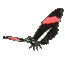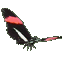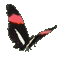Teaching Trigonometry Cosine Functions - Fun Math Help - Game Tips:

The cosine of angle A can be written as cos A and
cos A equals the ratio (x/r) = (3)/(5) when point D is (x,y) = (3,4) and r=AD=5.

With angle A in standard position at the origin, the cosine ratio of A is (x coordinate)/(radius) = (3)/(5).
The radius r=5 can be calculated from point D(3,4) using 32 + 42 = r2.

- When angle A is in standard position at the graph's origin (0,0) then cos A equals (x/r).
Since r is the hypotenuse of a right triangle, r can be found from point D(x,y) using x2 + y2 = r2 .

Consequently, cos A can also be described as (adjacent side)/(hypotenuse).
cos(A) = a/h = x/r .

- Your Game Score is reduced by the number of butterfly hits.

- To slow the game speed repeat tap/click on the word Slider.
- To increase the game speed repeat tap/click on the word Math.
- Speed can also be adjusted with a keyboard's - and + keys.

- Refresh/Reload the web page to restart the game.